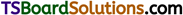# TS Inter 1st Year Chemistry Notes Chapter 4 States of Matter: Gases and Liquids

Here students can locate TS Inter 1st Year Chemistry Notes 4th Lesson States of Matter: Gases and Liquids to prepare for their exam.

## TS Inter 1st Year Chemistry Notes 4th Lesson States of Matter: Gases and Liquids

→ Parameters of gases are pressure, volume, temperature and number of moles.

→ Boyle’s law: It states that “at constant temperature, the volume of a given mass of a gas is inversely proportional to its pressure”.

→ Charles’ law : It states that “at constant pressure, the volume of a given mass of a gas is directly proportional to its absolute temperature”.

→ Avogadro’s law: It states that “at constant temperature and pressure, equal volumes of all gases contain equal number of molecules or moles”.

→ A gas which obeys Boyle’s law, Charles’ law and Avogadro’s law is called ideal gas.

→ Ideal gas equation is PV = nRT.

→ R is universal gas constant which denotes work done by a gas.

→ The equation of state is represented as
$$\frac{\mathrm{P}_1 \mathrm{~V}_1}{\mathrm{~T}_1}=\frac{\mathrm{P}_2 \mathrm{~V}_2}{\mathrm{~T}_2}$$

→ A real gas behaves as an ideal gas at high temperature and low pressure.

→ Diffusion is a phenomenon of intermixing of gases irrespective of gravitational force of attraction.

→ Effusion is diffusion through a small hole from a high pressure to low pressure area.→ Graham’s law of diffusion : It states that “at constant pressure and temperature the rate of diffusion of gas is inversely proportional to the square root of its density”.

→ Dalton’s law of partial pressure: It states that “the total pressure of a gaseous mixture which do not react chemically with each other is equal to the sum of the partial pressures of the component gases”.

→ Partial pressure = Total pressure × Mole fraction.

→ The pressure exerted by water vapour over liquid water surface when both of them are in equilibrium is called water vapour pres¬sure or aqueous tension.

→ Kinetic gas equation is given by PV = $$\frac{1}{3}$$mnc2.

→ Kinetic energy of a gas is equal to $$\frac{3}{2}$$ nRT.

→ The velocity possessed by maximum number of molecules is called the most probable velocity.

→ The ratio of sum of the velocities to the total number of molecules is called average velocity.

→ Square root of average for the squares of the velocities is called RMS velocity.

→ RMS velocity represents all gas molecules and is used in deriving kinetic gas equation.

→ The ratio of the three types of velocities is given as Cp : C̅ : C = 1 : 1.128 :1.224.

→ Gas constant per molecule is called
Boltzmann constant (K = $$\frac{\mathrm{R}}{\mathrm{N}}$$).

→ The method of separation of a mixture of two gases making use of the difference in their relative rates of diffusion or effusion is called atmolysis.